# Short Note Effect of velocity uncertainty on amplitude information

Robert G. Clapp

bob@sep.stanford.edu

Risk assessment is a key component to any business decision. Geostatistics has recognized this need and has introduced methods, such as simulation, to attempt to assess uncertainty in their estimates of earth properties Isaaks and Srivastava (1989). Geophysics has been slower to recognize this need, as methods which produce a single solution have long been the norm.

The single solution approach has a couple of significant drawbacks. First, since least-squares estimates invert for the minimum energy/variance solution, our models tend to have lower spatial frequency than the true model. Second, it does not provide information on model variability or provide error bars on the model estimate. Geostatisticians have both of these abilities in their repertoire through what they refer to as multiple realizations'' or stochastic simulations.'' They introduce a random component, based on properties of the data, such as variance, to their estimation procedure. Each realization's frequency content is more representative of the true model's and by comparing and contrasting the equiprobable realizations, model variability can be assessed. These models are often used for non-linear problems, such as fluid flow. In this approach representative realizations are used as an input to a flow simulator.

In geophysics we have a similar non-linear relationship between velocity and migration amplitudes. Migration amplitudes are used for rock property estimates yet we normally don't assess how velocity uncertainty, and the low frequency nature of our velocity estimates, affect our migration amplitudes. The geostatistical approach is not well suited to answer this question. Our velocity covariance is highly spatially variant, and our velocity estimation problem is non-linear.

In previous works Clapp (2000, 2001a,b), I showed how we can modify standard geophysical inverse techniques by adding random noise into the model styling goal to obtain multiple realizations. In this paper I apply this methodology to a conventional velocity analysis problem. I then migrate the data with various velocity realization. I perform Amplitude vs. Angle (AVA) analysis on each migrated image. Finally, I calculate the mean and variance of the AVA parameter estimates for the various relations. In this paper I review the operator based multi-realization methodology. I then apply the methodology on a structural simple 2-D land dataset from Columbia. MODEL VARIANCE

We can characterize the standard geophysical problem as a linear relationshipbetween a model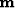and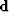, with a regularization operator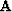. In terms of fitting goals this is:(1)
Ideallyshould be the inverse model covariance. If so, given an accurate modeling operator we would expect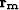to be zero. In fact,is an approximation of the inverse model covariance. In practice, we usually assume stationarity, and designto accurately describe the second order statistics of the model. The first order statistics, the spatial variance of the model, are not included. We can produce models that have similar spatial variance as the true model by modifying the second goal. This is done by replacing the zero vectorwith standard normal noise vector, scaled by some scalar,(2)
For the special case of missing data problems, whereis simply a masking operatordelineating known and unknown points, Claerbout (1998) showed howcan be approximated by first estimated model through the fitting goals in (1). Then, by solving,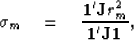(3)
whereis a vector composed of 1s. This basically says that we can find the right level of noise by looking at the residual resulting from applying our inverse covariance estimate on known data locations. If we make the assumption thatis accurate we can use (3) for a more general case. In the more general case, the operator is 1 at locations where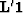is non-zero.